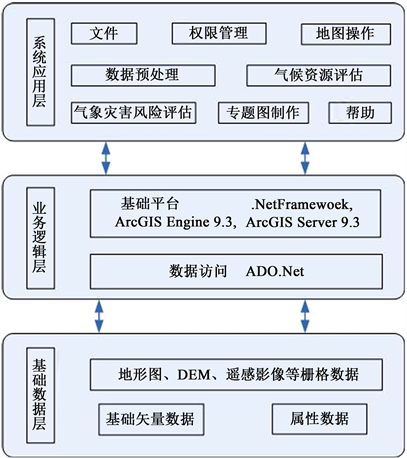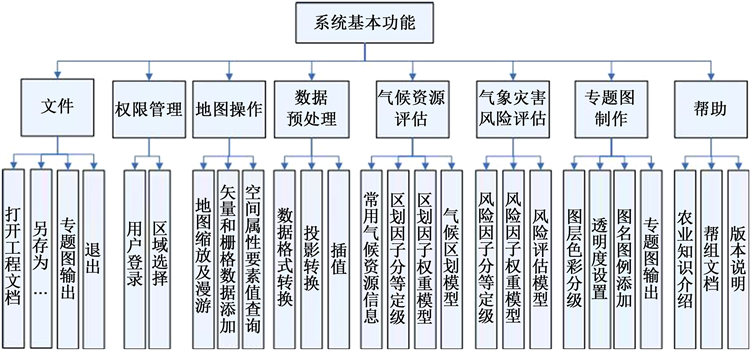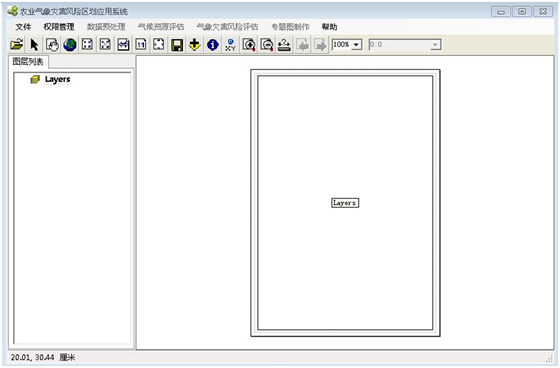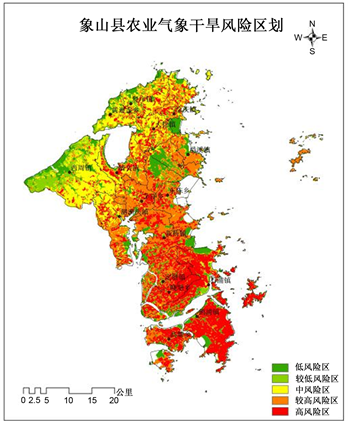# 基于GIS的农业气象灾害风险区划应用系统Application System of Agricultural Meteorological Disaster Risk Zoning Based on GIS

DOI: 10.12677/SEA.2020.96058, PDF, HTML, XML, 下载: 104  浏览: 196

Abstract: Agricultural meteorological disaster risk zoning is not only an important basis for rational use of natural resources, disaster prevention, disaster preparedness and insurance claims, but also plays an important role in optimizing agricultural structure, making disaster prevention and mitigation decisions and drawing social and economic development planning. The application system of ag-ricultural meteorological disaster risk zoning adopts C/S architecture. On the basis of absorbing and learning a lot of the latest research results of computer, spatial information processing, fuzzy mathematics theory and natural disaster and risk theory, the secondary development function package provided by ArcGIS Engine 9.3 is introduced to develop the system. With the support of high-resolution geographic information data, the system introduces component object technology, spatial interpolation technology, weight analysis technology and risk assessment technology to realize fine grid agricultural meteorological disaster risk zoning. Taking Xiangshan County agri-cultural meteorological drought risk zoning as an example, the application example shows that the system has a good applicability in agricultural meteorological disaster risk zoning.

1. 引言

2. 研究方法和主要功能

2.1. 研究方法

2.2. 系统整体架构介绍Figure 1. Basic system framework

2.2.1. 基础数据层

2.2.2. 业务逻辑层

2.2.3. 系统应用层

2.3. 系统基本功能Figure 2. Basic system functions

2.3.1. 文件

2.3.2. 权限管理

2.3.3. 地图操作

2.3.4. 数据预处理

2.3.5. 气候资源评估

2.3.6. 气象灾害风险评估

2.3.7. 专题图制作

2.3.8. 帮助

3. 关键技术

3.1. 组件对象技术

COM是Microsoft组件对象模型(Component Object Model)的简称，它与具体的编程语言和平台无关 。在COM构架下，人们可以开发出各种各样的功能专一的组件，然后将它们按照需要组合起来，构成复杂的应用系统。Microsoft的许多技术，如ActiveX, DirectX以及OLE等都是基于COM而建立起来的。这种做法的好处是可以随着技术的不断发展而用新的组件取代以有的组件。此时的应用程序可以随新组件不断取代旧的组件而渐趋完善。而且利用已有的组件，用户还可以快速的建立全新的应用。

3.2. 空间插值技术

3.2.1. 反距离权重插值

$z\left({x}_{j}\right)=\underset{i=1}{\overset{n}{\sum }}z\left({x}_{i}\right){d}_{ij}^{-r}/\underset{i=1}{\overset{n}{\sum }}{d}_{ij}^{-r}$ (1)

3.2.2. 克里金插值

$\stackrel{^}{z}\left({x}_{0}\right)=\underset{i=1}{\overset{n}{\sum }}{\lambda }_{i}z\left({x}_{i}\right)$ (2)

$E\left[\stackrel{^}{z}\left(x\right)-z\left(x\right)\right]=0$ (3)

$Var\left[\stackrel{^}{z}\left(x\right)-z\left(x\right)\right]\to \mathrm{min}$ (4)

(3)式和(4)式成立的话，则要求权重 ${\lambda }_{i}$ 要满足式(5)和式(6)：

$\underset{i=1}{\overset{n}{\sum }}{\lambda }_{i}\gamma \left({x}_{i},{x}_{j}\right)+\mu =\gamma \left({x}_{i},{x}_{0}\right)$ (5)

$\underset{i=1}{\overset{n}{\sum }}{\lambda }_{i}=1$ (6)

3.3. 权重分析技术

3.3.1. 层次分析法

1) 建立递阶层次结构模型；

2) 构造出各层次中的所有判断矩阵；

3) 层次单排序及一致性检验；

4) 层次总排序及一致性检验。通过以上四个步骤，最终得出每个指标的权重，以确定不同指标对同一因子的相对重要性。

3.3.2. 模糊数学评价法

1) 建立隶属函数

$f\left(u\right)=\left\{\begin{array}{l}0,u\le c\\ \frac{1}{1+{\left[a\left(u-c\right)\right]}^{b}},u>c\end{array}$ (7)

$a=\frac{\sqrt{99}}{{u}_{\mathrm{max}}-{u}_{\mathrm{min}}}$ (8)

2) 确定评价矩阵

$T=\left[\begin{array}{c}{T}_{1}\\ {T}_{2}\\ ⋮\\ {T}_{m}\end{array}\right]=\left[\begin{array}{cccc}{r}_{11}& {r}_{12}& \cdots & {r}_{1n}\\ {r}_{21}& {r}_{22}& \cdots & {r}_{2n}\\ ⋮& ⋮& & ⋮\\ {r}_{m1}& {r}_{m2}& \cdots & {r}_{mn}\end{array}\right]=\left[\begin{array}{cccc}{f}_{11}& {f}_{12}& \cdots & {f}_{1n}\\ {f}_{21}& {f}_{22}& \cdots & {f}_{2n}\\ ⋮& ⋮& & ⋮\\ {f}_{m1}& {f}_{m2}& \cdots & {f}_{mn}\end{array}\right]$ (9)

3) 风险评价因子权重的确定

$\mathrm{max}\left\{b\left(w\right)=\left({b}_{1}\left(w\right),{b}_{2}\left(w\right),\cdots ,{b}_{n}\left(w\right)\right)|\underset{i=1}{\overset{p}{\sum }}{w}_{i}^{2}=e\right\}$ (10)

${w}_{i}=d\frac{\underset{j=1}{\overset{n}{\sum }}{r}_{ij}}{\underset{i=1}{\overset{p}{\sum }}\underset{j=1}{\overset{n}{\sum }}{r}_{ij}}$$i=1,2,\cdots ,p$(11)

3.4. 风险评估技术

$\text{DRI}=\left({H}^{{W}_{H}}\right)\left({E}^{{W}_{E}}\right)\left({V}^{{W}_{V}}\right)\left[0.1\left(1-a\right)R+a\right]$ (12)

$H=\sum {W}_{Hk}{X}_{Hk}$

$E=\sum {W}_{Ek}{X}_{Ek}$

$V=\sum {W}_{Vk}{X}_{Vk}$

$R=\sum {W}_{Rk}{X}_{Rk}$

4. 系统应用实例Figure 3. The client interface on which the system runsFigure 4. Distribution map of agricultural meteorology drought risk division in Ningbo

5. 结语和讨论

  黄崇福. 自然灾害风险评价理论与实践[M]. 北京: 科学出版社, 2005.  葛全胜, 邹铭, 郑景云. 中国自然灾害风险综合评估初步研究[M]. 北京: 科学出版社, 2008.  陈升孛, 张亚杰, 张明洁, 等. 屯昌县蜜柚精细化农业气象灾害风险区划[J]. 热带农业科学, 2019, 39(8): 93-98.  王祥, 淮建军. 基于三级分层框架的农业气象灾害多风险评估[J]. 自然资源学报, 2020, 35(6): 1460-1471.  Hori, T., Zhang, J.Q., Tatano, H., et al. (2002) Micro-Zonation-Based Flood Risk Assessment in Urbanized Floodplain. Proceedings of the Second Annual IIASA-DPRI Meeting Integrated Disaster Risk Management, Vol. 1, 120-126.  黄丹萍, 白龙. 南宁城市内涝分布特征及其监测预警系统应用分析[J]. 防灾科技学院学报, 2019, 21(4): 84-89.  李爽爽. 精细化暴雨监测预报及风险预警系统的开发与应用[J]. 浙江气象, 2020, 43(3): 29-35.  兰腾达. 宁德市地质灾害气象风险预警系统应用与展望[J]. 福建地质, 2020(2): 155-160.  杨秀章. COM技术内幕——微软组件对象模型[M]. 北京: 清华大学出版社, l999.  彭勇刚, 黄肖寒, 莫益江, 等. 基于层次分析法的农业气象灾害风险区划指标权重分析[J]. 气象研究与应用, 2018, 39(1): 70-72.  周丽芸, 赵祈溶, 贺晨骋. 基于斜坡单元的皂市水库稳定性评价[J]. 测绘与空间地理信息, 2020, 43(10): 126-129+134.  支询, 林中冠, 沙莉, 等. 川南地区1989-2019年农业干旱危险性特征分析[J]. 农学学报, 2020, 10(8): 78-82.  胡波, 丁烨毅, 何利德, 等. 基于模糊综合评价的宁波暴雨洪涝灾害风险区划[J]. 暴雨灾害, 2014, 33(4): 380-385.  李登峰, 程春田, 陈守煜. 部分信息不完全的多目标决策方法[J]. 控制与决策, 1998, 13(1): 83-86.  张继权, 李宁. 主要气象灾害风险评价与管理的数量化方法及其应用[M]. 北京: 北京师范大学出版社, 2007.  Gary, S. (1997) An Assessment of Disaster Risk and Its Management in Thailand. Disaster, 2, 77-88. https://doi.org/10.1111/1467-7717.00045  胡波, 严甲真, 丁烨毅, 等. 台风灾害风险区划模型[J]. 自然灾害学报, 2012, 21(5): 152-158.